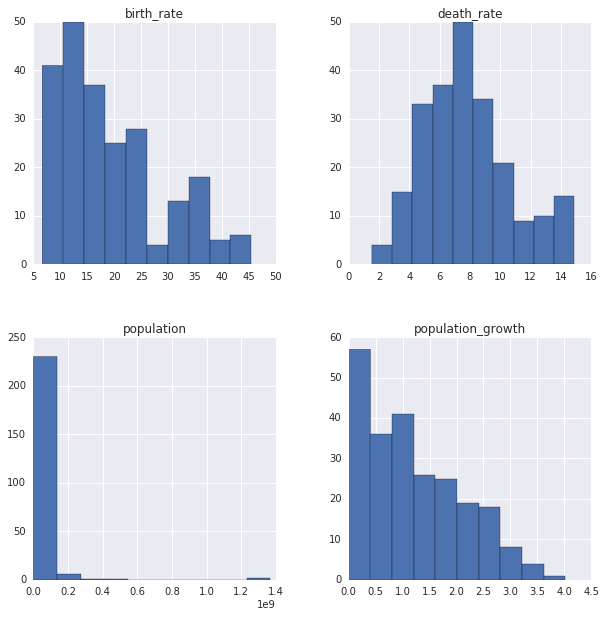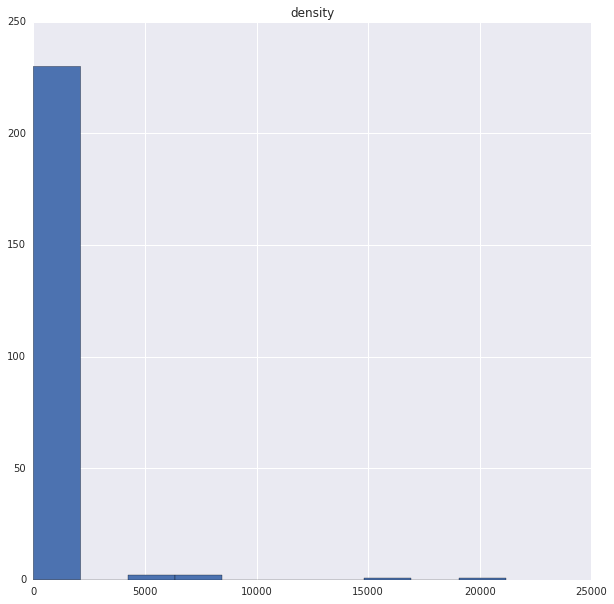# CIA World Factbook Analysis¶

In :
import sqlite3
import pandas as pd

conn = sqlite3.connect('factbook.db')

def run_query(query, conn = conn):

query_1 = "SELECT * FROM sqlite_master WHERE type='table';"
run_query(query_1)

Out:
type name tbl_name rootpage sql
0 table sqlite_sequence sqlite_sequence 3 CREATE TABLE sqlite_sequence(name,seq)
1 table facts facts 47 CREATE TABLE "facts" ("id" INTEGER PRIMARY KEY...
In :
query_2 = "SELECT * FROM facts LIMIT 5;"
run_query(query_2)

Out:
id code name area area_land area_water population population_growth birth_rate death_rate migration_rate
0 1 af Afghanistan 652230 652230 0 32564342 2.32 38.57 13.89 1.51
1 2 al Albania 28748 27398 1350 3029278 0.30 12.92 6.58 3.30
2 3 ag Algeria 2381741 2381741 0 39542166 1.84 23.67 4.31 0.92
3 4 an Andorra 468 468 0 85580 0.12 8.13 6.96 0.00
4 5 ao Angola 1246700 1246700 0 19625353 2.78 38.78 11.49 0.46

# Summary of Population and Growth¶

In :
query_3 = '''select min(population) min_pop, max(population) max_pop,
min(population_growth) min_pop_grwth, max(population_growth) max_pop_grwth
from facts'''
run_query(query_3)

Out:
min_pop max_pop min_pop_grwth max_pop_grwth
0 0 7256490011 0.0 4.02

# Lets deep dive in¶

In :
query_4  = '''
select * From facts where population == (select min(population) from facts)
'''
run_query(query_4)

Out:
id code name area area_land area_water population population_growth birth_rate death_rate migration_rate
0 250 ay Antarctica None 280000 None 0 None None None None
In :
query_5 = '''
select * from facts where population == (select max(population) from facts)
'''
run_query(query_5)

Out:
id code name area area_land area_water population population_growth birth_rate death_rate migration_rate
0 261 xx World None None None 7256490011 1.08 18.6 7.8 None

# Histograms of World¶

In :
import matplotlib.pyplot as plt
import seaborn as sns
%matplotlib inline

fig = plt.figure(figsize=(10,10))

query_6 = '''
select population, population_growth, birth_rate, death_rate
from facts
where population != (select max(population) from facts)
and population != (select min(population) from facts);
'''

/dataquest/system/env/python3/lib/python3.4/site-packages/IPython/core/interactiveshell.py:2885: UserWarning:

To output multiple subplots, the figure containing the passed axes is being cleared


Out:
array([[<matplotlib.axes._subplots.AxesSubplot object at 0x7f54e0502f98>,
<matplotlib.axes._subplots.AxesSubplot object at 0x7f54e0035748>],
[<matplotlib.axes._subplots.AxesSubplot object at 0x7f54dff6b400>,
<matplotlib.axes._subplots.AxesSubplot object at 0x7f54dfec5c50>]],
dtype=object)# Top 20 Highly Densed Countries¶

In :
query_7 = '''
select name as 'Country', cast(population as float)/cast(area as float) density from facts order by density desc limit 20
'''
run_query(query_7)

Out:
Country density
0 Macau 21168.964286
1 Monaco 15267.500000
2 Singapore 8141.279770
3 Hong Kong 6445.041516
4 Gaza Strip 5191.819444
5 Gibraltar 4876.333333
6 Bahrain 1771.859211
7 Maldives 1319.640940
8 Malta 1310.015823
9 Bermuda 1299.925926
10 Sint Maarten 1167.323529
12 Guernsey 847.179487
13 Jersey 838.741379
15 Mauritius 656.777941
16 Taiwan 650.781712
17 Aruba 623.122222
18 Lebanon 594.682788
19 Saint Martin 588.037037

# Histogram for population Density¶

In :
fig = plt.figure(figsize=(10,10))

array([<matplotlib.axes._subplots.AxesSubplot object at 0x7f54df99f390>],
dtype=object)